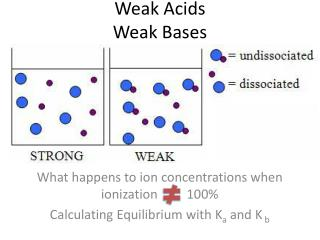DownloadDownload PresentationWeak Acids Weak Bases

# Weak Acids Weak Bases

Download Presentation## Weak Acids Weak Bases

- - - - - - - - - - - - - - - - - - - - - - - - - - - E N D - - - - - - - - - - - - - - - - - - - - - - - - - - -
##### Presentation Transcript

1. Weak AcidsWeak Bases What happens to ion concentrations when ionization 100% Calculating Equilibrium with Ka and K b

2. VIDEO REVIEW • View Acid Ionization Video Clip • View Base Ionization Video Clip How do weak acids and weak bases differ from strong?

3. Weak Acids

4. Ka= [H3O+] [A-] [HA] HA (aq) + H2O (l) A-(aq) + H3O+(aq) Weak or Partial Ionization Expression This equilibrium constant is called the acid-dissociation constant, Ka. Turn to p. 667, Table 16.2 ALSO in APPENDIX D

5. Cause of Acidic Behavior • Read p. 667- Last Sentence

6. Relevance + Magnitude of Ka The greater the value of Ka, the stronger is the acid. Kavalues for weak acids range from 10-2to 10-10.

7. [H3O+] [COO-] [HCOOH] Ka = Sample Exercise 16.10 p. 668 The pH of a 0.10 Msolution of formic acid, HCOOH, at 25C is 2.38. Calculate Ka for formic acid at this temperature. We know that To calculate Ka, we need the equilibrium concentrations of all three things.

8. 1. Use given pH to find [H+] pH = -log [H+] 2.38 = -log [H+] -2.38 = log [H+] 10-2.38 = 10log [H+] = [H+] 4.2  10-3 = [H+]

9. [4.2  10-3] [4.2  10-3] [0.10] Ka = 2. Set up ICE Chart 3. PLUG into Ka expression = 1.8  10-4

10. [H+]eq [HA]initial 4.2  10-3 0.10 Percent Ionization • Another measure of acid strength • The stronger the acid, the greater the percentage ionization • Percent Ionization =  100 • From previous example: [H+]eq = 4.2  10-3 M [HCOOH]initial~ 0.10 M  100 = 4.2%

11. Using Ka to calculate pH • Sample Exercise 16.12 p. 671

12. Weak Bases Bases react with water to produce hydroxide ion.

13. [HB] [OH-] [B-] Kb = Base Equilibrium Expression where Kb is the base-dissociation constant. Kb can be used to find [OH-] and, through it, pH.

14. Weak Bases

15. NH3(aq) + H2O (l) NH4+ (aq) + OH-(aq) [NH4+] [OH-] [NH3] = 1.8  10-5 Kb = Sample Exercise 16. What is the pH of a 0.15 M solution of NH3?

16. = 1.8  10-5 = [NH4+] [OH-] [NH3] Kb = (x)2 (0.15) 2. Use ICE method to tabulate data. (1.8  10-5) (0.15) = x2 2.7  10-6 = x2 1.6  10-3 = x2

17. 3. PLUG into pH equations [OH-] = 1.6  10-3 M pOH = -log (1.6  10-3) pOH= 2.80 pH = 14.00 - 2.80 pH = 11.20

18. KaandKb Relationship Kaand Kb are related in this way: Ka Kb = Kw Therefore, if you know one of them, you can calculate the other.